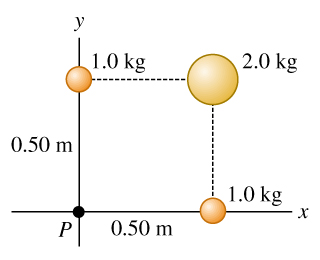# Problem: Three uniform spheres are fixed at positions shown in the figure.a) What is the magnitude of the force on a 0.0150-kg particle placed at point Pexttip{P}{P}?b) What is the direction of this force?c) If the spheres are in deep outer space and a 0.0150-kg particle is released from rest 300 m from the origin along a line 45° below the -x-axis, what will the particles speed be when it reaches the origin?

###### FREE Expert Solution

From Newton's law of universal gravitation, the attraction force between two masses M and m is given by:

$\overline{){\mathbf{F}}{\mathbf{=}}\frac{\mathbf{G}\mathbf{M}\mathbf{m}}{{\mathbf{r}}^{\mathbf{2}}}\stackrel{\mathbf{^}}{\mathbf{r}}}$###### Problem Details

Three uniform spheres are fixed at positions shown in the figure.
a) What is the magnitude of the force on a 0.0150-kg particle placed at point P?
b) What is the direction of this force?
c) If the spheres are in deep outer space and a 0.0150-kg particle is released from rest 300 m from the origin along a line 45° below the -x-axis, what will the particles speed be when it reaches the origin?